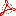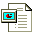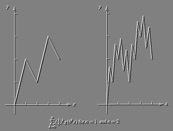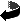MATH 554 - Math 703 I
Analysis I

Homework Assignments and Supplementary Materials

To view an Acrobat PDF version of each Solution Set, click on the symbol.
(Free Acrobat reader can be found here.)
The iconindicates a PowerPoint file.

Homework Assignments
(Each Problem is worth 10 pts.)

 No. Assignment Due Page and No.'s Solutions #1 Wednesday (1/21) In problems 1-2, assume F is a field: Carefully prove that multiplicative inverses are unique. Carefully prove that (-1)(-1)=1. You can use any propositions which we have proved in class. In Z5, what is the (i) additive inverse of 1; (ii) the multiplicative inverse of 3, of 4; (iii) find the solution of the equation 3 x + 1 =2.   (Hint: Make addition and multiplication tables and do look-ups.)#2 Friday (1/30) Suppose F is an ordered field. If an element a in F satifies |a|< d for each d>0, then carefully prove that a=0. Page 30 of text: 4(a); 5 (a), (b), (c); 6.; 10 (a), (b) Extra Credit: 10 (c) Additional Graduate Student Problems: Page 30: 11#3 Friday (2/06) Prove the equivalence statement for "greatest lower bounds" (we proved in class the similar statement for least upper bounds): If L is the greatest lower bound for a set A, then   (i)   L is a lower bound for A   (ii)   if e>0, then there exists a in A so that a < L+e. Prove that if L1 and L2 are both least upper bounds for a set A, then L1=L2. (Moral: least upper bounds are unique) Using the property that the real numbers satisfy the completeness axiom (i.e. the least upper bound property), prove that R satisfies the property that each nonempty set B, which is bounded from below, must have a greatest lower bound.   (Hint: Consider the negatives of sets and the fact that inequalities are reversed when multiplied by a negative.) Using induction, prove that 1+r+r2+ ... + rn = (1-rn+1)/(1-r) if r is not equal to 1. Using the properties of "<", prove that the interval     (a,b) = {x in R |   |x-c|< r} where c=(a+b)/2 and r=(b-a)/2.#4 Friday (2/13) Click here to download the assignment.#5 Monday (2/23) Click here to download the assignment. Extra Credit (15 pts.)-- Prove the following about closure of sets: Given a set S, let C be S unioned with all the limit points of S. Show that the complement of C is open. (Hence C is closed.) C is called the "closure" of S. Suppose that D is a closed set containing S, then prove that the closure of S is contained in D. Prove that the intersection of all closed sets containing S is the same set as the closure of S. MainE.C.#6 Friday (2/27) Problem:   Suppose that S has the property that each sequence from S which converges, must converge to a limit which belongs to S. Prove that S is a closed set. Extra Credit (10 pts.)-- Complete the proof of the following: Suppose in the real numbers the sequence {bn } converges to b and b is not zero. Prove that 1/bn converges to 1/b.#7 Friday (3/26) You can download homework assignment #7 for Friday March 26, here.#8 Friday (4/9) Problem:   Using properties of connectedness and the last theorem proved in Monday's lecture (about continuity of inverse functions), prove that for each natural number n: non-negative n-th roots of non-negative numbers exist and furthermore, the function g(x) := x1/n is continuous for x larger or equal zero. Extra Credit (10 pts.)-- Fill in the details of the proof begun during Monday's lecture: Prove that a function is continuous if and only if the inverse image of a closed set is closed. Be sure to prove the set equality relating inverse images of the complements of sets.This page maintained by Robert Sharpley (sharpley@math.sc.edu) and last updated Nov. 2, 2003.  This page ©2003-2004, The Board of Trustees of the University of South Carolina.  URL: http://www.,math.sc.edu/~sharpley/math554

Return to Math 554 Home Page